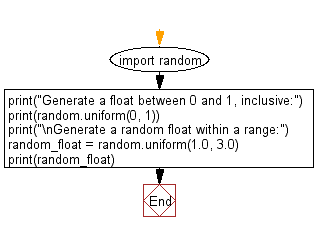﻿ Python: Generate a random float within a specific range - w3resource# Python: Generate a random float within a specific range

## Python module: Exercise-7 with Solution

Write a Python program to generate a float between 0 and 1, inclusive and generate a random float within a specific range.

Use random.uniform

Sample Solution:

Python Code:

``````import random
print("Generate a float between 0 and 1, inclusive:")
print(random.uniform(0, 1))
print("\nGenerate a random float within a range:")
random_float = random.uniform(1.0, 3.0)
print(random_float)
```
```

Sample Output:

```Generate a float between 0 and 1, inclusive:
0.9332552133311842

Generate a random float within a range:
1.3166101982165992
```

Flowchart:## Visualize Python code execution:

The following tool visualize what the computer is doing step-by-step as it executes the said program:

Python Code Editor:

Have another way to solve this solution? Contribute your code (and comments) through Disqus.

What is the difficulty level of this exercise?

Test your Python skills with w3resource's quiz

﻿

## Python: Tips of the Day

For-else construct useful when searched for something and find it:

```# For example assume that I need to search through a list and process each item until a flag item is found and
# then stop processing. If the flag item is missing then an exception needs to be raised.

for i in mylist:
if i == theflag:
break
process(i)
else:
raise ValueError("List argument missing terminal flag.")
```